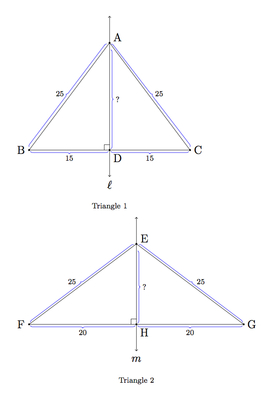# Two Triangles' Area

Alignments to Content Standards: 8.G.B.7

Let $A$ be the area of a triangle with sides of length 25, 25, and 30. Let $B$ be the area of a triangle with sides of length 25, 25, and 40. Find $A/B$.

## IM Commentary

This task requires the student to draw pictures of the two triangles and also make an auxiliary construction in order to calculate the areas (with the aid of the Pythagorean Theorem). Students need to know, or be able to intuitively identify, the fact that the line of symmetry of the isosceles triangle divides the base in half, and meets the base perpendicularly. Since reflective symmetry belongs to the 8th grade standards, it may be reasonable to assume this knowledge, or the teacher may wish to help students with this part of the picture if they are struggling. Once the student has the appropriate pictures with the perpendicular bisectors drawn the rest of the problem is an application of the Pythagorean theorem.

This task was adapted from problem #16 on the 2011 American Mathematics Competition (AMC) 8 Test. For the 2011 AMC 8, which was taken by 153485 students, the multiple choice answers for the problem had the following had the following distribution:

 Choice Answer Percentage of Answers (A) ${A=\frac{9}{16}B}$ 13.80 (B) ${A=\frac{3}{4}B}$ 44.92 (C)* ${A=B}$ 13.43 (D) ${A=\frac{4}{3}B}$ 15.04 (E) ${A=\frac{16}{9}B}$ 5.46 Omit - 7.28

Of the 153485 students: 72648, or 47% were in 8th grade; 50433, 72648, or 33% were in 7th grade; and the remainder were less than 7th grade.

## Solution

We begin by drawing pictures of the two triangles. These triangles are both isosceles because they each have a pair of sides whose length is 25. An isosceles triangle has a single line of symmetry and this is shown in the picture below:The line of symmetry of an isosceles triangle divides the base in half, and meets it at a right angle: this allows us to fill in the lengths of the different segments in the pictures, leaving the two altitudes $\overline{AD}$ and $\overline{EH}$ to determine. For triangle 1, we can apply the Pythagorean Theorem in order to calculate $|AD|$: $$|AD|^2 + |BD|^2 = |AB|^2.$$ Plugging in the known values $|BD| = 15$ and $|AB| = 25$ we find $|AD|^2 = 400$ so $|AD| = 20$. This also tells us that $|EH| = 15$ because $\triangle ABD$ and $\triangle FEH$ are both right triangles whose hypotenuse is 25 and each has a leg measuring 15 so the other leg must be 20 by the Pythagorean theorem. Thus we see that $\triangle ABC$ has the same area as $\triangle EFG$: for $\triangle ABC$ we have $|BC| = 30$ and $|AD| = 20$ giving an area of $\frac{1}{2} \times 30 \times 20 = 300$ and for $\triangle EFG$ we have $|FG| = 40$ and$|EH| = 15$ giving an area of $\frac{1}{2} \times 40 \times 15 = 300$.

In fact the final calculation of areas is not necessary: since both $\triangle ABC$ and $\triangle DEF$ are broken into pairs of right triangles with the same side lengths they must have the same area.